How to use the CHISQ.TEST function in Excel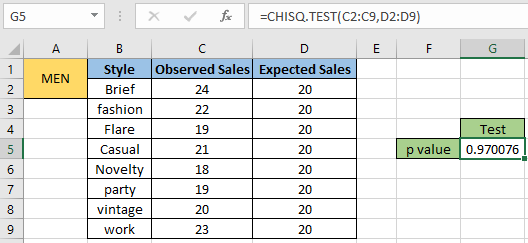In this article, we will learn How to use the CHISQ.TEST function in Excel.

What is Chi square test?

The Chi Square test is very important as many test statistics are distributed as Chi Square distribution. Chi Square distributions are tests of independence between theoretical expection and observed frequencies.

A chi-squared test (also written as x2), is a statistical hypothesis test that is valid to perform when the test statistic is chi-squared distributed under the null hypothesis. It can be used to find out the variations in observed and expected frequencies, e.g., defective items produced by machines A and B. Comparing the observed and expected frequencies with this function will help us understand if sampling error caused the difference in the two frequencies. Mathematically, Chi square distribution (x2) is calculated using the formula shown below.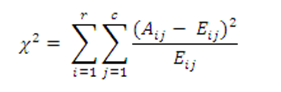Here :

Aij – Actual frequency in the i’th row and j’th column

Eij – Expected frequency in the i’th row and j’th column

r – Number of rows

c – Number of columns

Chi square distribution is also based on the degree of freedom for the given dataset. And how does it depend on it? Now you must be wondering what is the degree of freedom (also denoted as k). Mathematically, the degree of freedom(k) of a distribution is equal to the number of standard normal deviations being summed. See how degrees of freedom (k) affect the chi square density distribution in the different plots shown below.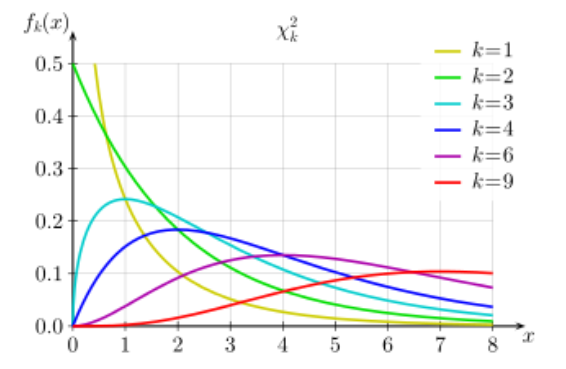In the above plot we can see the p value variations caused by the degree of freedom k. Let's learn how to use the CHI SQUARE test using the CHISQ.TEST function to calculate the relation between the two given datasets (actual and observed).

CHISQ.TEST Function in Excel

The CHISQ.TEST function in Excel returns the chi-squared probability of something across datasets. The function takes the actual dataset and expected dataset as argument and returns the probability relation between the two.

CHISQ.TEST Function syntax:

 =CHISQ.TEST(actual_range , expected_range)

actual_range : actual range or observed range

expected_range : expected range before the actual range

Example :

All of these might be confusing to understand. Let's understand how to use the function using an example. Here we have a sample dataset of dress styles worn by men. So the manufacturing company decided to measure the p value relation between the observed sales and actual sales. Here sales are in thousands.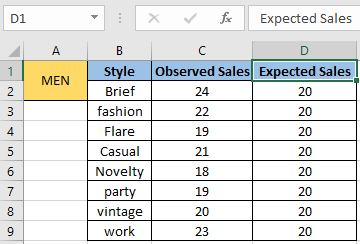Now for the given chi square distributed dataset we need to find the relation using the formula stated below.

Use the formula:

 =CHISQ.TEST(C2:C9,D2:D9)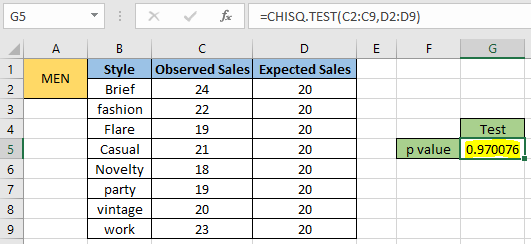As you can see the test returned around 0.97 or 97% relation between the two, it means the observed sales is close to the expected sales result. The test returning value above 0.5 shows the good relation and indicates the level of dependence between the two whereas the test returning value less than 0.5 is an indicator of independence.

Now let's consider one more case for the actual and observed sales for women style wear.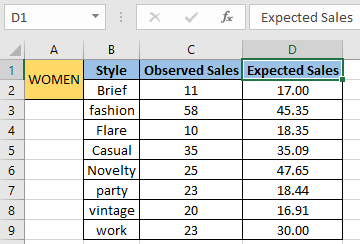Now for the given chi square distributed dataset we need to find the relation using the formula stated below.

Use the formula:

 =CHISQ.TEST(C2:C9,D2:D9)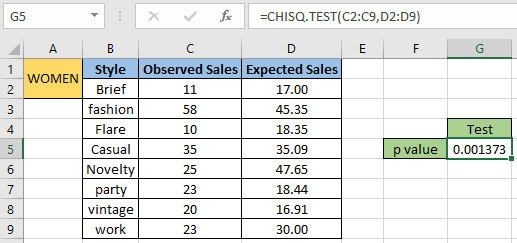The p value for the calculated by chi square test comes out to be 0.0014 which is even less than 1. This means observed sales didn't go according to the expected sales.

Here are all the observational notes using the CHISQ.TEST function in Excel
Notes :

1. The function only works with numbers. If any argument other than cumulative is non numeric, the function considers the non numeric value as 0 and returns the result.
2. Value in decimal or value in percentage is the same value in Excel. Convert the value to percentage, if required.
3. The function returns #N/A error if the length of the two range arguments is not equal or the dataset contains only one value i.e the length and width equal to 1.
4. The function returns #DIV/0 error if any of the values provided in expected_range is zero.
5. The function returns #NUM! Error, if any number in range is negative.

Hope this article about How to use the CHISQ.TEST function in Excel is explanatory. Find more articles on statistical formulas and related Excel functions here. If you liked our blogs, share it with your friends on Facebook. And also you can follow us on Twitter and Facebook. We would love to hear from you, do let us know how we can improve, complement or innovate our work and make it better for you. Write to us at info@exceltip.com.

Related Articles :

How To Use Excel T TEST Function in Excel : The T.TEST is used to determine the confidence of an analysis. Mathematically, it is used to know if the mean of the two samples are equal or not. T.TEST is used to accept or reject the null hypothesis.

How to use Excel F.TEST Function in Excel : The F.TEST Function is used to calculate F statistic of two samples in excel internally and returns the two tailed probability of the F statistic under Null Hypothesis.

How to use the DEVSQ Function in Excel : DEVSQ function is a built-in statistical function to calculate the sum of squared deviations from the mean or average of the range of data values provided.

How to use Excel NORM.DIST Function : Calculate the Z score for the normal cumulative distribution for the pre specified values using the NORMDIST function in Excel.

How to use Excel NORM.INV Function : Calculate the inverse of Z score for the normal cumulative distribution for the pre-specified probability values using the NORM.INV function in Excel.

How to Calculate Standard Deviation in Excel : To calculate the standard deviation we have different functions in Excel. The standard deviation is the square root of the variance value but It tells more about the dataset than variance.

How to use the VAR function in Excel : Calculate the variance for the sample dataset in excel using the VAR function in Excel.

Popular Articles :

How to use the IF Function in Excel : The IF statement in Excel checks the condition and returns a specific value if the condition is TRUE or returns another specific value if FALSE.

How to use the VLOOKUP Function in Excel : This is one of the most used and popular functions of excel that is used to lookup value from different ranges and sheets.

How to use the SUMIF Function in Excel : This is another dashboard essential function. This helps you sum up values on specific conditions.

How to use the COUNTIF Function in Excel : Count values with conditions using this amazing function. You don't need to filter your data to count specific values. Countif function is essential to prepare your dashboard.

Terms and Conditions of use

The applications/code on this site are distributed as is and without warranties or liability. In no event shall the owner of the copyrights, or the authors of the applications/code be liable for any loss of profit, any problems or any damage resulting from the use or evaluation of the applications/code.